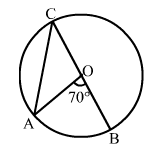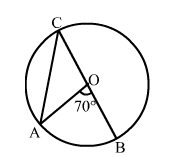# In the given figure, O is the canter of the circle and ∠AOB = 70°.

Question:

In the given figure, O is the canter of the circle and AOB = 70°.
Calculate the values of (i) ∠OCA, (ii) ∠OAC.Solution:(i)

The angle subtended by an arc of a circle at the centre is double the angle subtended by the arc at any point on the circumference.

Thus, AOB = 2OCA

$\Rightarrow \angle O C A=\left(\frac{\angle A O B}{2}\right)=\left(\frac{70^{\circ}}{2}\right)=35^{\circ}$

(ii)

OA = OC  (Radii of a circle)
OAC = OCA    [Base angles of an isosceles triangle are equal]

$=35^{\circ}$